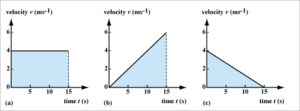# Motion Along a Straight Line

0
3231In nature, we rarely encounter uniform movement. Usually, the bodies change the speed of movement during their movement.

Before starting to move, the speed of the car is zero. At the beginning of the movement this speed increases. When approaching the destination, the car reduces speed and ultimately stops. All living beings move at different speeds. In the picture we can see the tiger as he chases the antelope constantly increasing his speed over time in order to catch it.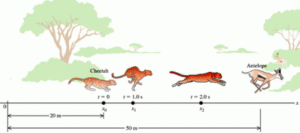The movement in our example is changing, because the speed changes over time.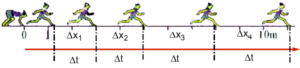The picture shows the position of the girl during the same time interval. We see that for the same time intervals the path traveled is longer. The positions Δx1, Δx2, Δx3 and Δx4 show that Δx1 <Δx2 <Δx3 <Δx4 for girl increases her velocity. The velocity changes over time.

In the example above the velocity is increasing, and in the picture below on the left, we see an increase in velocity over time, but also that the distances boy crosses become shorter, which means his velocity is being reduced.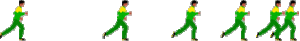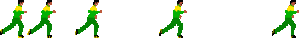A movement at which the rate of velocity changes with respect to time is called acceleration.

Acceleration

Acceleration is equal to velocity per unit of time. This physical size is denoted by a. If the instantaneous velocity of the body is v, and the initial velocity is vo, the movement occurs in the time interval Δt = t-to, then the acceleration is given by the relation: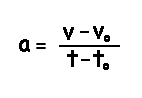The acceleration unit is one meter per second for a second, or 1 m / s²

The body has a unit acceleration if every second its velocity increases for it one meter per second.

If the velocity change is the same for all subsequent time intervals, we say that the body moves with constant acceleration. Thus, a straight uniformly changed motion is the movement in which the acceleration is constant. a = const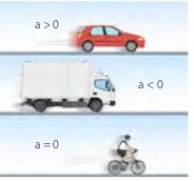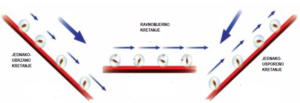If the acceleration is positive (a> 0), the body increases the velocity over time, and if the acceleration is negative (a <0) the body decreases the speed over time (slows down).

Acceleration with uniformly changed motion does not change over time, so we can take any time interval to calculate the velocity. Let the initial velocity of movement of the car be equal to zero vo = 0. This means that the car was quiet before the start of the movement. The car starts to accelerate evenly. By definition, acceleration is: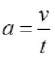Since the initial velocity is zero (vo = 0), the instantaneous speed of the car after time t can be written in the form above. The velocity of the car uniformly increases with time.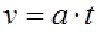If the body has an initial velocity vo, then the velocity is: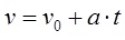The speed can be calculated by: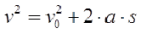or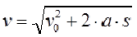Crossed road with uniformly changing straight line movement

The path of any changeable movement can be found by medium speed, because: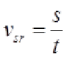In the case of uniform straight-line motion with the initial velocity, the path is: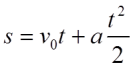if vo = 0, the length of the travelled path is: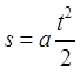Current velocity of uniformly accelerated movement with initial speed: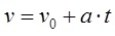Current velocity of uniformly decelerated movement: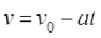Current velocity of uniformly accelerated movement without initial velocity:Length of traveled path of uniformly accelerated movement with initial speed: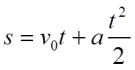Length of traveled path of uniformly decelerated movement: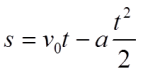The length of the path of uniformly accelerated movement without initial velocity: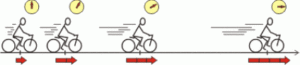Example; Calculate the acceleration of the bicyclist, if his velocity at the start of the movement was zero, and the current velocity 30m / s, in 15 minutes.

vo = 0

v = 30m / s

t = 15min = 15.60s = 60s

__________________

Task: Calculate the acceleration using the given sizes from the image: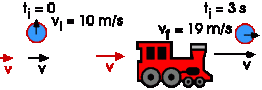Roadmap and the velocity of equal uniformly accelerated movement

As with a uniform and even uniformly accelerated motion, we can represent it graphically. The velocity chart of uniformly accelerated movement, without starting speed is called a v – t diagram.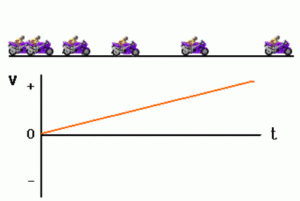Graph of velocity, time, and acceleration in a uniformly changeable motion as the function of time: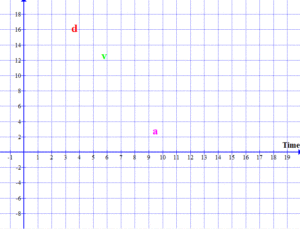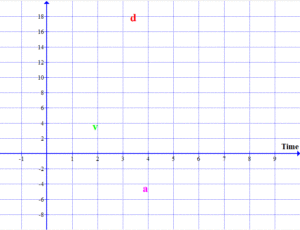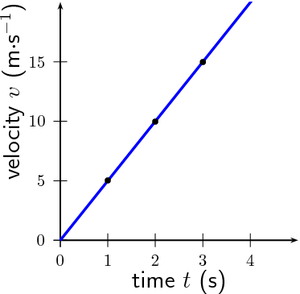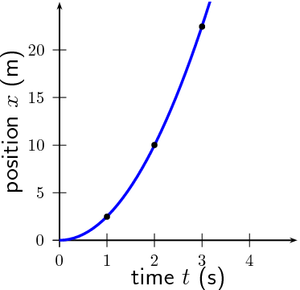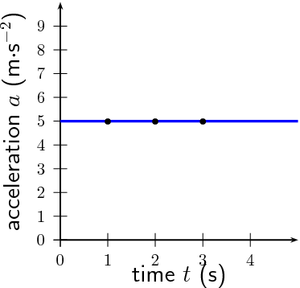With a uniformly variable straight line motion, the velocity changes, while the acceleration has a constant value of a = const, and the acceleration graph is parallel with the axis of time.